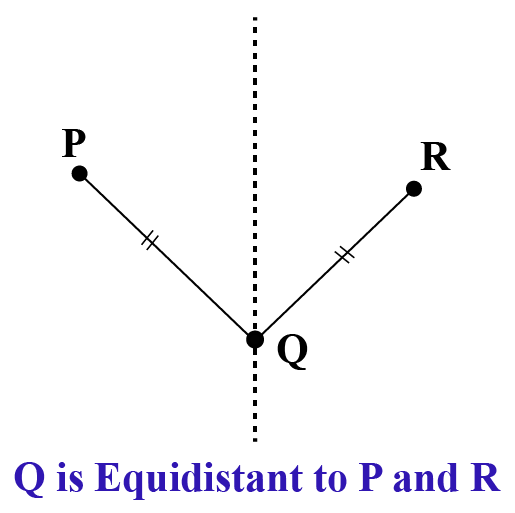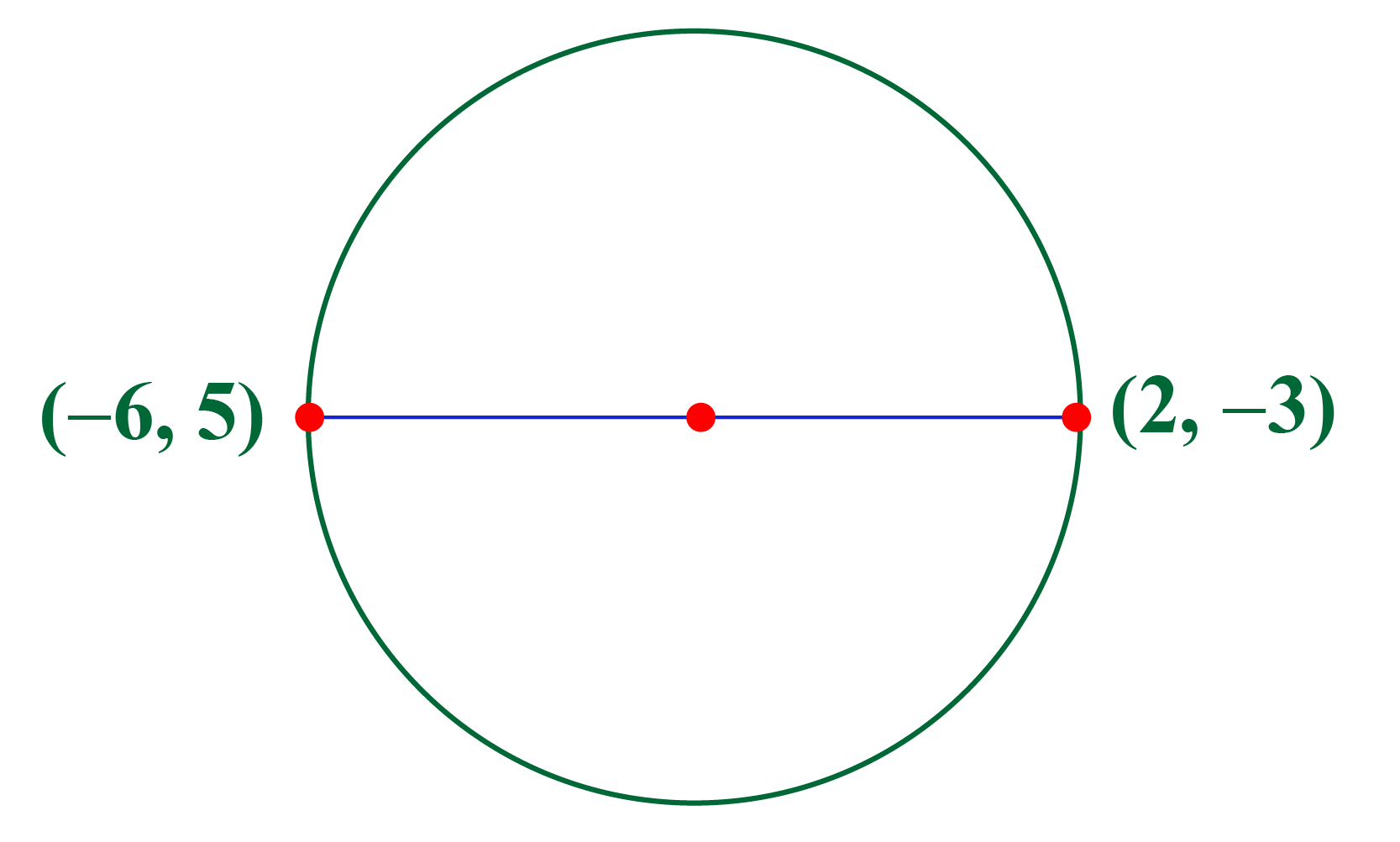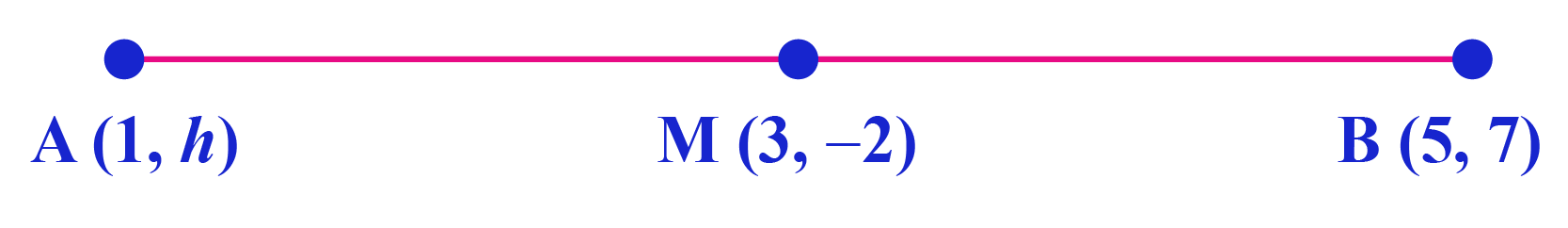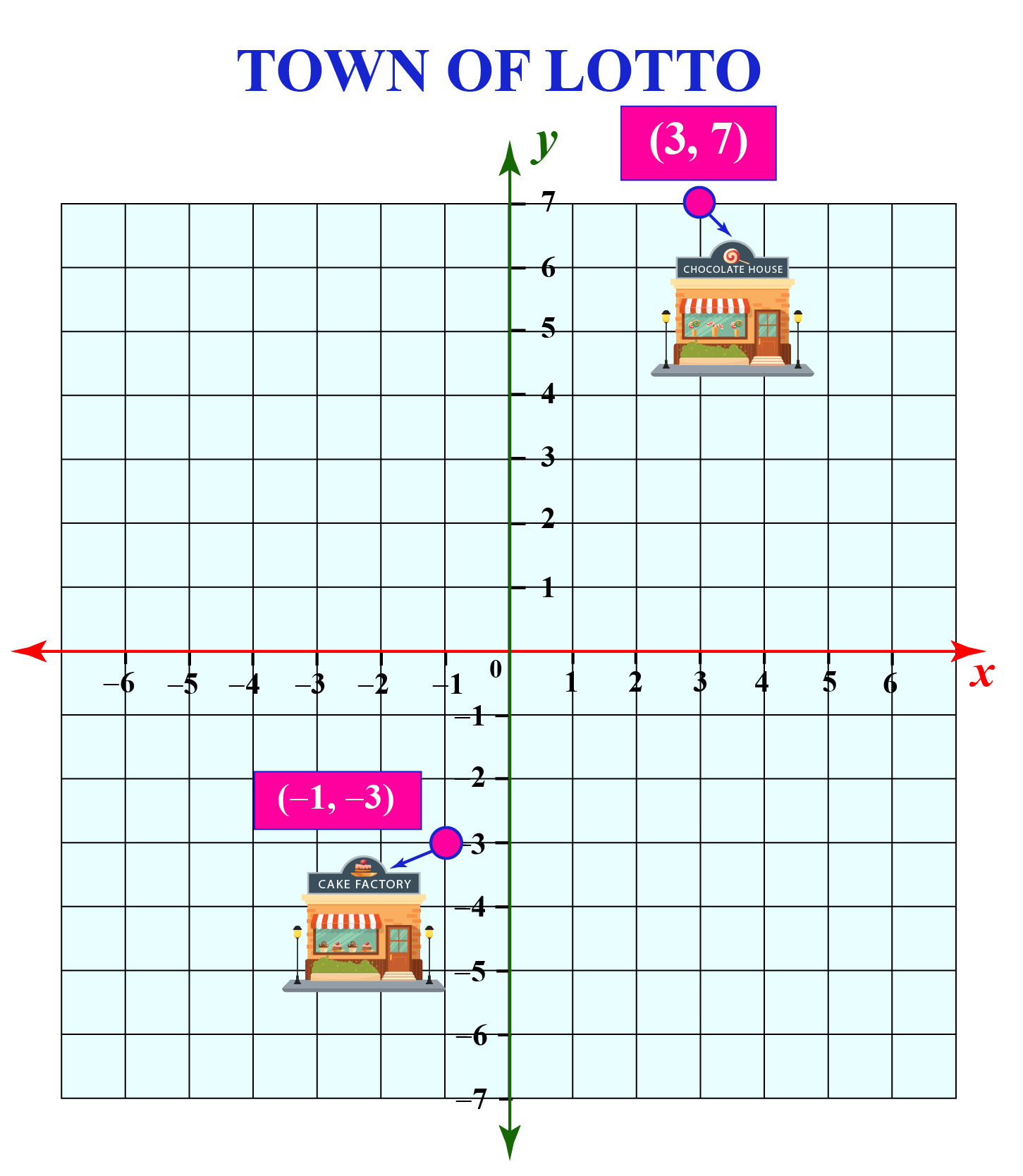# Equidistant

In this mini-lesson, we will explore the world of equidistant. We will do so by learning the definition of equidistant, its general formula, how to calculate it, the role of the midpoint, and other interesting facts around the concept.

If we take points P and R, then from the below diagram, we can say that point Q is equidistant from two points P and R.Before we get started, did you know, the first use of the concept "Equidistant" was used in the year, 1556?

Actually, this word is derived from late Latin - aequi + distans meaning "of equal distance" from two points.

## What Is the Definition of Equidistant?

A point is said to be equidistant from other points if it is always distanced equally or of the same distance away from each point.

Equidistant meaning in maths is mostly used in geometry, in the concepts of parallel lines/planes, perpendicular bisectors, circles, angle bisectors, etc.

In the illustration shown earlier, we can also say that Point Q is the midpoint to points P and R.

## What Is the General Formula of Equidistant?

Generally, to find the equidistance for any two objects or points, we use both the midpoint formula and distance formula

### Midpoint Formula

When two points A ($$a$$, $$b$$) and B ($$p$$, $$q$$) are given, the midpoint, which is equidistant from each end will be

 $M = (\frac {a+p}{2}, \frac {b+q}{2})$

### Distance Formula

Considering the same points as above, the distance, d  between these two points will be

 $d = \sqrt{(p - a^2) + (q - b)^2 }$

Have a look at the equidistant calculator here:

Try entering any two points and see what trajectory do its equidistant points show!Think Tank

Find the $$x$$, if point A (x, 8) is equidistant from point C (6, 4) and B (12,12)

Is it possible to get 2 such points A? Can you justify your statement?

## Solved Examples

 Example 1

Find a point such that it lies on the y-axis and is equidistant from the points $$(-1, 2$$) and $$(2, 3)$$

Solution

We know that the x-coordinate of any point on the y-axis is $$0$$

Hence, we assume the point that is equidistant from the given points to be $$(0, k$$), i.e.

Distance between $$(0, k)$$ and $$(-1, 2)$$ $$=$$ Distance between $$(0, k)$$ and $$(2, 3)$$

\begin{aligned} \!\!\sqrt{(\!\!-\!1\!-\!0\!)^2+\!(\!2\!-\!k\!)^2}\!=\! \sqrt{(\!2-0\!)^2+(\!3-k\!)^2}\\ \end{aligned}

Squaring on both sides

\begin{aligned}(-1-0)^2+(2-k)^2 &=(2-0)^2+(3-k)^2 \\1 + 4+k^2-4k &= 4+ 9 +k^2-6k \\2k &=8 \\ k&=4 \end{aligned}

Therefore, the required point is, $$(0, k)=(0, 4)$$

 $$\therefore$$ Required Point  $$= (0, 4)$$
 Example 2

The diameter of a circle has endpoints, (2, -3) and (-6, 5).Can you find the coordinates of the center of this circle?

Solution

The center of a circle divides the diameter into 2 equal parts.

So, it is a midpoint of the diameter.

Let $$x_{1}=2$$, $$y_{1}=-3$$, $$x_{2}=-6$$, and $$y_{2}=5$$

The coordinates of the center is calculated as:

\begin{align}\left(\dfrac{x_{1}+x_{2}}{2}, \dfrac{y_{1}+y_{2}}{2}\right)&=\left(\dfrac{2+(-6)}{2}, \dfrac{-3+5}{2}\right)\\&=\left(-\dfrac{4}{2}, \dfrac{2}{2}\right)\\&=\left(-2,1\right)\end{align}

 $$\therefore$$ The center of the circle is $$(-2,1)$$
 Example 3

Consider the line segment $$\overline{AB}$$ shown below.The points $$A=(1, h)$$ and $$B=(5, 7)$$ are equidistant from their midpoint M.

Find the value of $$h$$ if the midpoint of $$\overline{AB}$$ is $$(3, -2)$$

Solution

Let $$x_{1}=1$$, $$y_{1}=h$$, $$x_{2}=5$$, and $$y_{2}=7$$

According to the definition of midpoint we have,

\begin{align}\left(\dfrac{x_{1}+x_{2}}{2}, \dfrac{y_{1}+y_{2}}{2}\right)&=(3,-2)\\\left(\dfrac{1+5}{2}, \dfrac{h+7}{2}\right)&=(3,-2)\\\dfrac{h+7}{2}&=-2\\h+7&=-4\\h&=-11\end{align}

 $$\therefore$$ The value of $$h=-11$$
 Example 4

The town of Lotto is mapped on a coordinate plane as shown.The chocolate house is located at the point (3, 7) and the cake factory is located at the point (-1, -3)

The main entrance of the town is located on the x-axis but is at equal distance from the chocolate house and the cake factory.

Can you calculate the point of the main entrance?

Solution

To calculate the point of the main entrance, we have to calculate the midpoint of (3, 7) and (-1, -3)

Let $$x_{1}=3$$, $$y_{1}=7$$, $$x_{2}=-1$$, and $$y_{2}=-3$$.

The coordinates of the main entrance is calculated as:

\begin{align}\left(\dfrac{x_{1}+x_{2}}{2}, \dfrac{y_{1}+y_{2}}{2}\right)&=\left(\dfrac{3+(-1)}{2}, \dfrac{7+(-3)}{2}\right)\\&=\left(\dfrac{2}{2}, \dfrac{4}{2}\right)\\&=\left(1, 2\right)\end{align}

 $$\therefore$$ The main entrance is located at the point (1, 2)

More Important Topics
Numbers
Algebra
Geometry
Measurement
Money
Data
Trigonometry
Calculus